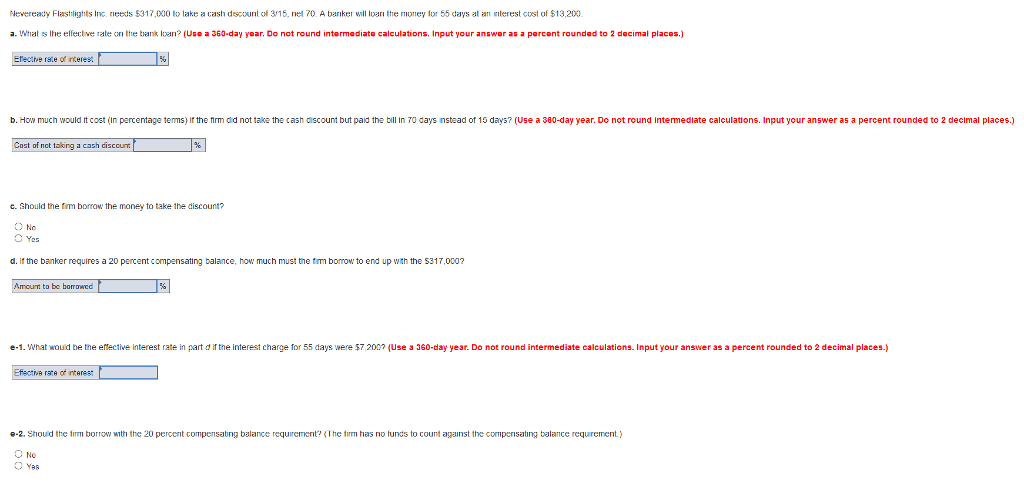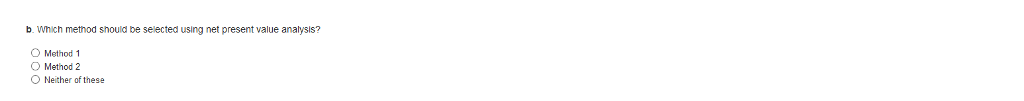# Neveready Flashlights Inc Needs 317 000 To Take A Cash Discount Of 3 15 Net 70 A Ban 2457403

Neveready Flashlights Inc. needs \$317,000 to take a cash discount of 3/15, net 70. A banker will loan the money for 55 days at an interest cost of \$13,200.

a. What is the effective rate on the bank loan? (Use a 360-day year. Do not round intermediate calculations. Input your answer as a percent rounded to 2 decimal places.)

b. How much would it cost (in percentage terms) if the firm did not take the cash discount but paid the bill in 70 days instead of 15 days? (Use a 360-day year. Do not round intermediate calculations. Input your answer as a percent rounded to 2 decimal places.)

c. Should the firm borrow the money to take the discount?

No Yes

d. If the banker requires a 20 percent compensating balance, how much must the firm borrow to end up with the \$317,000?

e-1. What would be the effective interest rate in part d if the interest charge for 55 days were \$7,200? (Use a 360-day year. Do not round intermediate calculations. Input your answer as a percent rounded to 2 decimal places.)

e-2. Should the firm borrow with the 20 percent compensating balance requirement? (The firm has no funds to count against the compensating balance requirement.)

No YesNeveready Flashlights Inc. needs \$317,000 to lake a cash discount of 315, nel 70. A bariker will loarn the money for 55 days at an interest cost of \$13,200. a. Wat is the elfective rale on the brank loan? (Use a 360-day year, Do not round intermediate calculations. Input your answer as a percent rounded to 2 decimal places.) Eecive rate of interes alculations b. How much would t cost (in percentage tems) ir the nrm did not taike the cash discount but paid the bill in 70 days instead ot 15 days? (Use a 380-day year. Do not round intermediate calculations. Input your answer as a percent rounded to 2 decimal places. Coct of nct takirg a cash dixceurt ? cash discount c. Should the fm bomow the money to take the discount? money to scount? O No Yes d. It the banker requires a 20 percent compansating balance, how much must the tim borrow to end up wth the 5317,000? orrow to to bc bomawcd e-1. What wouid be te effective interest rate in part d if the interest charge for 55 days were \$7,200 (Use a 360-day year. Do not round intermediate calculations. Input your answer as a percent rounded to 2 decimal places.) 0-2. Should the firm . Should the firm borrow with the 20 percent compensating balance requrement?? he trm has no tunds to count against the compensating balance requireme unt against the No Yas